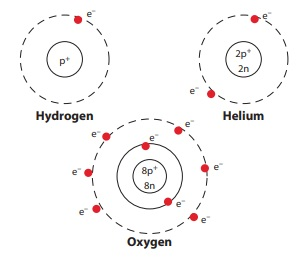Home | | Science 7th Std | Atomic number and Mass number

# Atomic number and Mass number

If all elements are made up of same type of electrons, protons and neutrons how does a carbon atom differ from a iron atom? Further investigations led to the discovery that the number of the protons inside the nucleus of an atom determines what element it is.

Atomic number and Mass number

If all elements are made up of same type of electrons, protons and neutrons how does a carbon atom differ from a iron atom? Further investigations led to the discovery that the number of the protons inside the nucleus of an atom determines what element it is. For Example if the nucleus has only one proton, then all such atoms are hydrogen atom. If there are eight protons then that atom is oxygen.

Is the structure of the atom the same as the structure of the solar system? Yes ! It is similar to the solar system. It has a core center called nucleus and it has paths called orbits around the nucleus.

Atomic number (z)

The number of electrons or protons in anatomis called the atomic number of that atom .It is represented by the letter Z. If we know the atomic number of an atom, we know the number of electrons or protons in it.Look at the figures. The hydrogen nucleus has one proton around which revolves one electron. It means that its atomic number z=1.

In the helium atom there are two protons and two electrons in orbit around the nucleus, so the atomic number of helium is z=2.

Look at the atomic structure of oxygen shown in the figure. What is its atomic number?

Try yourself

If the atomic number of carbon is Z=6, what is the number of the electrons revolving in its atom

Mass number (A) or Atomic mass :

We have seen that the mass of an atom is concentrated in its nucleus. From this, we can get the atomic mass number. mass number (A) is equal to the sum of the number of protons(p) and neutrons (n) in the nucleus.

Automic mass or mass number = Number of Protons + Number of Neutrons

A = p+n

A lithium atom contains 3 Protons and 4 neutrons . Its atomic mass number A = 3+4 = 7.

In a sodium atom, there are 11 Protons and 12 neutrons. Hence , its atomic mass number A=11+12=23.

Try yourself

1. Why are atomic numbers and mass numbers are always whole numbers ?

2. A sulphur atom contains 16 Protons and 16 neutrons . Give its atomic number and atomic mass number.

When writing the symbol of an element, its atomic number and atomic mass number are also written. For example, the symbols of hydrogen, carbon and oxygen are written as 1H1, 6C12, 8O16 respectively.

All the elements in the periodic table have the following combination of protons, electrons and neutrons:Isotopes: Atoms of the same element can have different number of neutrons. Such atoms will have same atomic number but different mass numbers. These atoms are called isotopes. For example Hydrogen has three isotopes ---Hydrogen (1H1), Deuterium (1H2), Tritium (1H3).

Isobars: Atoms that have the same mass number but different atomic numbers. for example Calcium – 40 and Argon – 40

Elements and their symbols with their atomic number and mass number.ACTIVITY 3Observe the table given above and answer the following questions.

1. I am used for breathing, without me you cannot live. Do you know me? Write my name  and symbol Oxygen, O.

2. It is used in filling the balloons. It is a gas, identity it. What is its mass number? Hydrogen. (A=l).

3. Name the element present in banana. What is my atomic number? Potassium (Z = 19)

4. I am found in crackers. How many protons do i have? Sulfur (p = 16)

5. I am the most valuable element. Find who am I? Can you say my mass number? Carbon (A= 12)

Tags : Atomic Structure | Term 1 Unit 4 | 7th Science , 7th Science : Term 1 Unit 4 : Atomic Structure
Study Material, Lecturing Notes, Assignment, Reference, Wiki description explanation, brief detail
7th Science : Term 1 Unit 4 : Atomic Structure : Atomic number and Mass number | Atomic Structure | Term 1 Unit 4 | 7th Science